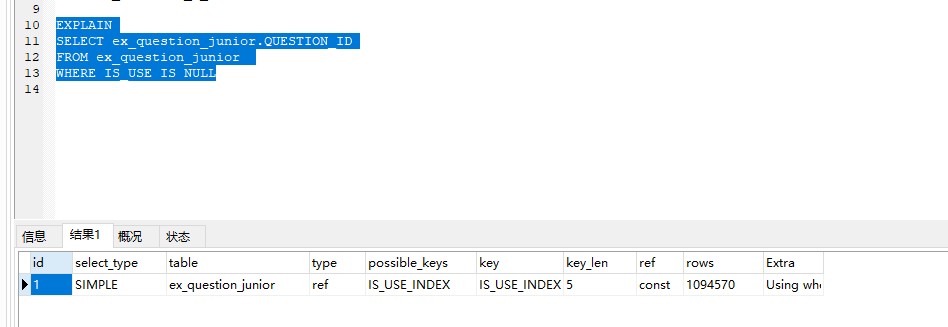## Problem

Using MySQL Connector/Python, you are calling a stored procedure which
is also selecting data and you would like to fetch the rows of the
result.

## 案例分析3：

1.
in()适合B表比A表数据大的情况（A<B）

select * from A

where id in(select id from B)

2.
exists()适合B表比A表数据小的情况(A>B)

select * from A

where exists(

select 1 from B where B.id =
A.id

)

3、当A表数据与B表数据一样大时,

in与exists效率差不多,可任选一个使用.语法

****************************************************************************

ex_question_r_knowledge(A)表数据量大，ex_subject_point表数据量小(B)(A>B)

``````SELECT *
FROM ex_question_r_knowledge
WHERE ex_question_r_knowledge.SUBJECT_POINT_ID IN
(
SELECT ex_subject_point.SUBJECT_POINT_ID
FROM ex_subject_point
WHERE ex_subject_point.SUBJECT_ID=7
)
``````

``````SELECT *
FROM ex_question_r_knowledge
WHERE  exists
(
SELECT 1
FROM ex_subject_point
WHERE ex_subject_point.SUBJECT_ID=7
AND ex_subject_point.SUBJECT_POINT_ID = ex_question_r_knowledge.SUBJECT_POINT_ID
)
``````

*************************************************************************

ex_subject_point表数据量小(A),ex_question_r_knowledge(B)表数据量大(A<B)

``````SELECT *
FROM ex_subject_point
WHERE ex_subject_point.SUBJECT_POINT_ID IN(
SELECT ex_question_r_knowledge.SUBJECT_POINT_ID
FROM ex_question_r_knowledge
``````

``````SELECT *
FROM ex_subject_point
WHERE  exists(
SELECT ex_question_r_knowledge.SUBJECT_POINT_ID
FROM ex_question_r_knowledge
AND ex_question_r_knowledge.SUBJECT_POINT_ID= ex_subject_point.SUBJECT_POINT_ID
)
``````

4.

# 2、应尽量避免在 where 子句中对字段进行 null 值判断，否则将导致引擎放弃使用索引而进行全表扫描

select id from t where num
is null

NULL填充数据库.

select id from t where num =
0

## Discussion

The `stored_results`() method
of cursor object is retiring an iterator object which will go over the
results proceeded after calling the stored procedure. Each result is
actually a MySQLCursorBuffered object.

You could also use
the `with_rows` cursor property
to check if the cursor actually could return rows or not (for example,
for SELECT statements). An example is provided in
the documentation.

## 案例分析：``````SELECT ex_question_junior.QUESTION_ID
FROM ex_question_junior
WHERE IS_USE is NULL
````````````SELECT ex_question_junior.QUESTION_ID
FROM ex_question_junior
WHERE IS_USE =0
``````

## Solution

For this example we create a stored procedure which is
executing `SHOW SLAVE STATUS`.

“`python cnx = mysql.connector.connect(user=’scott’,
cur.execute(“DROP PROCEDURE IF EXISTS slave_status”) proc = “CREATE
PROCEDURE slave_status () BEGIN SHOW SLAVE STATUS; END” cur.execute()
cur.call(“slave_status”)

for result_cursor in cur.stored_results(): for row in result_澳门微尼斯人手机版，cursor:
print(row) “`

The result from the above would be:

`shell \$ python foo.py Waiting for master to send event`

# 4.应尽量避免在 where 子句中使用 or 来连接条件，如果一个字段有索引，一个字段没有索引，将导致引擎放弃使用索引而进行全表扫描

Java开发环境，需要配置MySQL，按照Windows的习惯，使用Navicat for MySQL

## 案例分析：

``````SELECT ex_question_junior.QUESTION_ID
FROM ex_question_junior
``````

a、字段出现在查询条件中，并且查询条件可以使用索引；

b、语句执行频率高，一天会有几千次以上；

c、通过字段条件可筛选的记录集很小，那数据筛选比例是多少才适合？2.